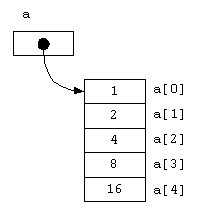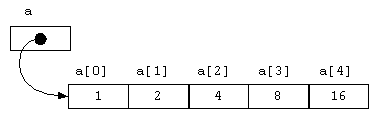# Arrays

Arrays are to data as loops are to control flow.

## Overview

The basic solution. Arrays are the basic way to store large numbers of values. Every programming language you're likely to use has arrays as part of the fundamental language, altho with many little variations on how they work.

Strings are like arrays of chars and you should be familiar with the idea of storing multiple values which are accessed by a numeric index.

Other possibilities. Arrays in Java are great for working with a fixed numbers of elements, but Java also has the Collections library of classes that are a better choice when working with a variable number of objects, eg, `ArrayList`, but it's essential to learn about arrays, so we'll start with them.

## Arrays store many values using one name and an index

An array can store many similar values in memory. Each value is accessed by specifying an integer subscript or index in brackets following the array name. "Array" in Java means approximately the same thing as array, matrix, or vector does in math. Unlike math and some other programming languages, in Java you must both declare an array and allocate a fixed amount of memory for it.

## Declaring an array

An array variable is like other variables -- you must declare it, which means you must declare the type of elements that are in an array. All elements must be the same type. Write the element type name, then "[]", then the name of the array variable. The declaration allocates only enough space for a reference to an array (typically 4 bytes), but doesn't create the actual array object.

```String[]  args;   // args is an array of Strings
int[]     scores; // scores is an array of ints
JButton[] controlButtons;  // controlButtons is an array of JButtons```

No size in declaration. Unlike some languages, never put the size of the array in the declaration because an array declaration specifies only the element type and the variable name. The size is specified when you allocate space for the array.

## Names - Plurals or collective nouns are most common for array names

Most programming guidelines suggest using plural names, or nouns denoting a collection of things, for arrays and other collections of multiple values. This is not a rigid rule, and your choice will often be based on linguistic sensitives. For example, you might have an array of words and their frequencies. It would be entirely appropriate to name this `wordFrequencyTable` because the word "table" suggests many entries. If you had an array of single words, you might call it `words` or `wordList`. Naming the array using the singular `word` would probably be very confusing to most readers.

Examples in this text often follow the common convention of using the array variable name "a". This seriously violates the rule of having meaningful names, so please don't adopt this textbook-example style in your code!

## Allocate an array object with `new`

Create an array using `new`. This example creates an array of 100 int elements, from `a` to `a`.

```int[] a;           // Declare a to be an array of ints
a = new int;  // Allocate an array of 100 ints```

These are often combined in one line.

`int[] a = new int;  // Declare and allocate.`

## Subscripts (indexes/indices)

Subscripts are enclosed in square brackets `[]`. `xi` in mathematics is `x[i]` in Java, and is pronounced "x-sub-i".

Subscript ranges always start at zero because Java came largely from C++, which had a good reason for using zero (pointer arithmetic on arrays). It isn't the way that humans normally count; you'll just have to live with it.

Java always checks subscript legality to be sure the subscript is >= 0, and less than the number of elements in the array. If the subscript is outside this range, Java throws `ArrayIndexOutOfBoundsException`. This is far superior to the behaver of C and C++, which allow out of range references. Consequently, Java programs are far less susceptible to bugs and security flaws than C/C++ programs.

Zero-based indexing is a constant annoyance of Java's zero-based indexing. The natural human value for hours or days should be used as an index to make the program most readable, but this would mean starting with 1, not zero.

Translate or ignore 0 element? If the data you are working with naturally starts at one, not zero, either the data must be modified before using it as an index, or the size of the array could be increased by one row so that the natural values could be used, and row 0 would never be referenced. Ignoring the zeroth array element instead of translating data to be zero based is a style decision, and you'll find various positions on this matter. See the AutoSales example below.

## Length of an array

Each array has a constant (`final`) instance variable that has its length. You can find out how many elements an array can hold by writing the array name followed by `.length`. In the previous example, `a.length` would be 100. Remember that this is the number of elements in the array, one more than the maximum subscript.

## Example - Reading into an array

This shows typical input code for reading into an array from a Scanner. This program is very strange because it doesn't do anything with the data, but it's shown here as an early step in the iterative process.

 ``` 1 2 3 4 5 6 7 8 9 10 11 12 13 14 15 16 17 18 19 20 21 22 23 24 ``` ```// InputArray.java -- Simple demo of reading into an array. // Fred Swartz - 26 Aug 2006 - Placed in the public domain. import java.util.*; public class InputArray { public static void main(String[] args) { Scanner in = new Scanner(System.in); int[] scores = new int; int n = 0; // Current number of values in scores. while (in.hasNextInt()) { if (n >= scores.length) { System.err.println("Too much data!"); System.exit(1); } scores[n] = in.nextInt(); n++; } //... Now do something with the scores! } } ```

## Java idiom for looping over an array - for loop

For loop. The most common use of `.length` is in a for loop test condition. For example, the variable `i` will go over the entire range of subscripts of the array `a`.

```int[] a = new int; // Declare, allocate array of 1000 ints, a...a

//... Assign random values to each element.
for (int i = 0; i < a.length(); i++) {
a[i] = (int)(Math.random() * 100000);  // Random number 0-99999
}
//... Add all elements of the array a.
int sum = 0;
for (int i = 0; i < a.length; i++) {
sum += a[i];
}```

Enhanced for loop. If you only need to reference the value of each of the elements, you can use the somewhat simpler enhanced for loop (also known as the foreach loop), which keeps track of the index and assigns successive values to a variable (`v` in this example). The foreach loop only gets the values, so it couldn't have been used to set the values in the first loop above.

```//... Add all elements of the array a.
int sum = 0;
for (int v : a) {
sum += v;
}```

## Initial array element values -- zero/null/false

When an array is allocated (with `new`), all elements are set to an initial value. The initial value is 0 if the element type is numeric (int, float, ...), `false` for boolean, and `null` for all object types.

## Array Initialization

When you declare an array, you can can also allocate a preinitialized array object in the same statement. In this case, do not give the array size because Java counts the number of values to determine the size. For example,

`String[] days = {"Su", "Mo", "Tu", "We", "Th", "Fr", "Sa"};`

## Array diagrams

This code declares and initializes an array.

`int[] a = {1, 2, 4, 8, 16};`

Arrays are often represented with diagrams that represent their memory use. The diagrams below are typical ways to represent the memory used by an array.

Each box represents the amount of memory needed to hold one array element. For ints this is 4 bytes. The array variable, a, is a reference (ie, memory address in the heap) of the block of memory that holds the actual array elements. References are often represented by a black disk with an arrow pointing to the data they reference. Most often array diagrams are written vertically, but sometimes the cells are arranged horizontally, especially for arrays of characters. Of course in the actual physical memory there is no such idea as vertical or horizontal.or## Array variables are references to a block of elements

When you declare an array variable, Java reserves only enough memory for a reference (Java's name for an address or pointer) to an array object. References typically require only 4 bytes. When an array object is created with `new`, a reference is returned, and that reference can then be assigned to a variable. When you assign one array variable to another, only the reference is copied. For example,

```int[] a = new int[] {100, 99, 98};  // "a" references the array object.
int[] b;                            // "b" doesn't reference anything.

b = a;      // Now "b" refers to the SAME array as "a"
b = 0;   // Also changes a because a and b refer to the same array.```

## Example (AutoSales) - Translating between subscript and data ranges

Indexes in other ranges. If the index data is in a range far from zero, eg auto sales by year, translate the index by subtracting the minimum value. For example,

 ``` 1 2 3 4 5 6 7 8 9 10 11 12 13 14 15 16 17 18 19 20 21 22 23 24 25 26 27 28 29 30 31 32 33 34 35 36 ``` ```// File : data-arrays/autoSales/AutoInfo.java // Purpose: Demonstrates use of arrays to hold values associated // with integer values that don't start at zero. // Author : Fred Swartz - 2006-01-15 - Placed in public domain. import java.util.*; public class AutoInfo { //======================================================== constants static final int FIRST_YEAR = 1900; static final int LAST_YEAR = 2020; //============================================================= main public static void main(String[] args) { int[] autoSales = new int[LAST_YEAR - FIRST_YEAR + 1]; Scanner in = new Scanner(System.in); //... Read data as pairs of year and sales values. while (in.hasNextInt()) { int year = in.nextInt(); int sales = in.nextInt(); autoSales[year - FIRST_YEAR] = sales; } //... Here's where code to process this data belongs. //... Print year and sales for all non-zero values. for (int year = FIRST_YEAR; year <= LAST_YEAR; year++) { int sales = autoSales[year - FIRST_YEAR]; if (sales > 0) { System.out.println(year + " " + sales); } } } } ```

Alternate loop to display values. Another way to write the above output is to loop over index values, then translate these to the appropriate data range.

```//... Print year and sales for all non-zero values.
for (int i = 0; i < autoSales.length; i++) {
int sales = autoSales[i];
int year  = FIRST_YEAR + i;
if (sales > 0) {
System.out.println(year + " " + sales);
}
}```

## Common array problems

Some common array programming mistakes are:
• Compile-time error: Writing `a.length()` instead of `a.length`. The `length()` method is used with Strings, not arrays.
• Compile-time error: Declaring an array with a size. Eg, `int a;` instead of `int[] a = new int;`.